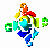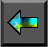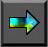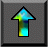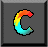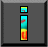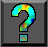Next: Chapter Notes Up: 8 Message Passing Interface Previous: 8.9 Summary

# Exercises

1. Devise an execution sequence for five processes such that Program 8.4 yields an incorrect result because of an out-of-order message.

2.   Write an MPI program in which two processes exchange a message of size N words a large number of times. Use this program to measure communication bandwidth as a function of N on one or more networked or parallel computers, and hence obtain estimates for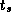and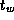.

3. Compare the performance of the program developed in Exercise 2 with an equivalent CC++ or FM program.

4.   Implement a two-dimensional finite difference algorithm using MPI. Measure performance on one or more parallel computers, and use performance models to explain your results.

5. Compare the performance of the program developed in Exercise 4 with an equivalent CC++ , FM, or HPF programs. Account for any differences.

6. Study the performance of the MPI global operations for different data sizes and numbers of processes. What can you infer from your results about the algorithms used to implement these operations?

7. Implement the vector reduction algorithm of Section 11.2 by using MPI point-to-point communication algorithms. Compare the performance of your implementation with that of MPI_ALLREDUCE for a range of processor counts and problem sizes. Explain any differences.

8.   Use MPI to implement a two-dimensional array transpose in which an array of size N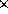N is decomposed over P processes ( P dividing N ), with each process having N/P rows before the transpose and N/P columns after. Compare its performance with that predicted by the performance models presented in Chapter 3.

9. Use MPI to implement a three-dimensional array transpose in which an array of size NNN is decomposed over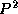processes. Each processor has (N/P)(N/P) x/y columns before the transpose, the same number of x/z columns after the first transpose, and the same number of y/z columns after the second transpose. Use an algorithm similar to that developed in Exercise 8 as a building block.

10.   Construct an MPI implementation of the parallel parameter study algorithm described in Section 1.4.4. Use a single manager process to both allocate tasks and collect results. Represent tasks by integers and results by real numbers, and have each worker perform a random amount of computation per task.

11. Study the performance of the program developed in Exercise 10 for a variety of processor counts and problem costs. At what point does the central manager become a bottleneck?

12. Modify the program developed in Exercise 10 to use a decentralized scheduling structure. Design and carry out experiments to determine when this code is more efficient.

13. Construct an MPI implementation of the parallel/transpose and parallel/pipeline convolution algorithms of Section 4.4, using intercommunicators to structure the program. Compare the performance of the two algorithms, and account for any differences.

14. Develop a variant of Program 8.8 that implements the nine-point finite difference stencil of Figure 2.22.

15. Complete Program 8.6, adding support for an accumulate operation and incorporating dummy implementations of routines such as identify_next_task.

16. Use MPI to implement a hypercube communication template (see Chapter 11). Use this template to implement simple reduction, vector reduction, and broadcast algorithms.Next: Chapter Notes Up: 8 Message Passing Interface Previous: 8.9 Summary